Скачать презентацию 1 Chapter One Chemistry Matter and Measurement Prentice

6d7e2a6150d368b16a44b0831652d9d4.ppt

• Количество слайдов: 38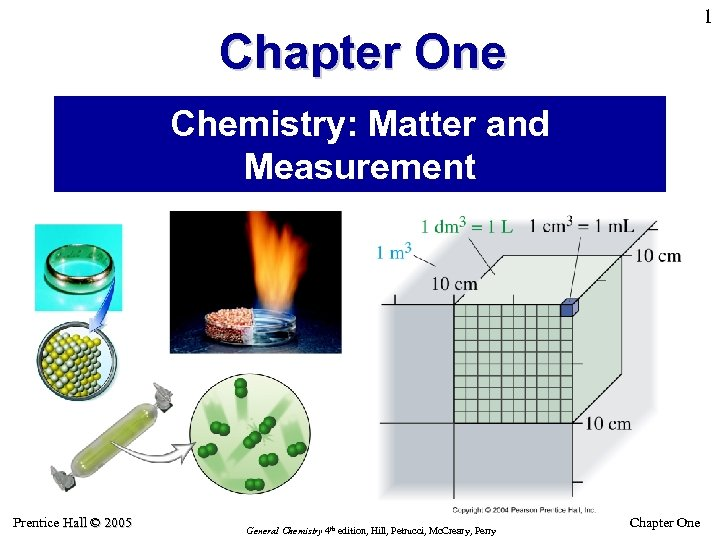1 Chapter One Chemistry: Matter and Measurement Prentice Hall © 2005 General Chemistry 4 th edition, Hill, Petrucci, Mc. Creary, Perry Chapter One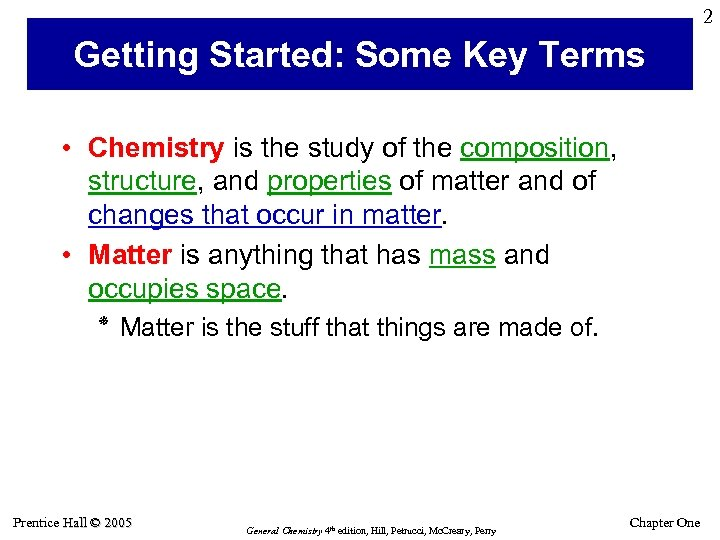2 Getting Started: Some Key Terms • Chemistry is the study of the composition, structure, and properties of matter and of changes that occur in matter. • Matter is anything that has mass and occupies space. ٭ Matter is the stuff that things are made of. Prentice Hall © 2005 General Chemistry 4 th edition, Hill, Petrucci, Mc. Creary, Perry Chapter One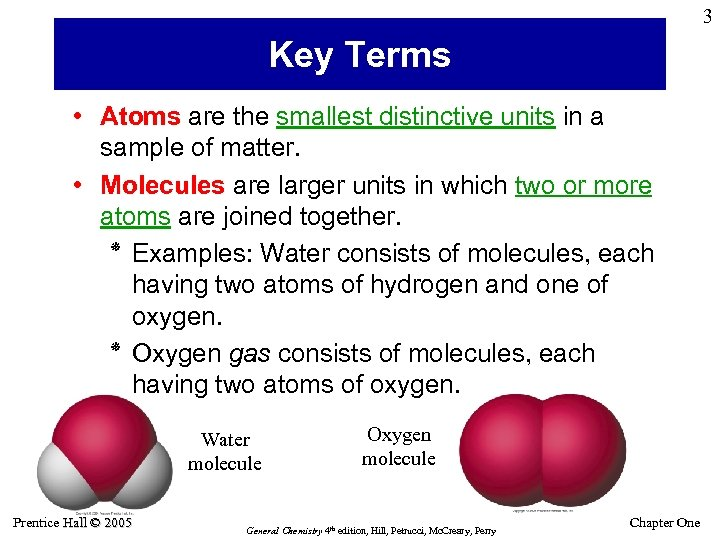3 Key Terms • Atoms are the smallest distinctive units in a sample of matter. • Molecules are larger units in which two or more atoms are joined together. ٭ Examples: Water consists of molecules, each having two atoms of hydrogen and one of oxygen. ٭ Oxygen gas consists of molecules, each having two atoms of oxygen. Water molecule Prentice Hall © 2005 Oxygen molecule General Chemistry 4 th edition, Hill, Petrucci, Mc. Creary, Perry Chapter One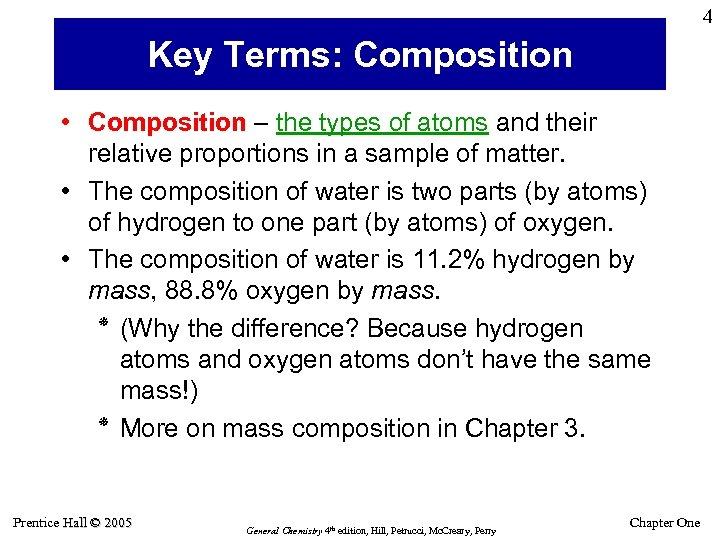4 Key Terms: Composition • Composition – the types of atoms and their relative proportions in a sample of matter. • The composition of water is two parts (by atoms) of hydrogen to one part (by atoms) of oxygen. • The composition of water is 11. 2% hydrogen by mass, 88. 8% oxygen by mass. ( ٭ Why the difference? Because hydrogen atoms and oxygen atoms don’t have the same mass!) ٭ More on mass composition in Chapter 3. Prentice Hall © 2005 General Chemistry 4 th edition, Hill, Petrucci, Mc. Creary, Perry Chapter One5 Key Terms: Properties • A physical property is displayed by a sample of matter without undergoing any change in the composition of the matter. ٭ Physical properties include mass, color, volume, temperature, density, melting point, etc. • A Chemical property is displayed by a sample of matter as it undergoes a change in composition. ٭ Flammability, toxicity, reactivity, acidity are all chemical properties. Prentice Hall © 2005 Copper is red-brown, opaque, solid: physical properties. Ethanol is flammable: a chemical property. General Chemistry 4 th edition, Hill, Petrucci, Mc. Creary, Perry Chapter One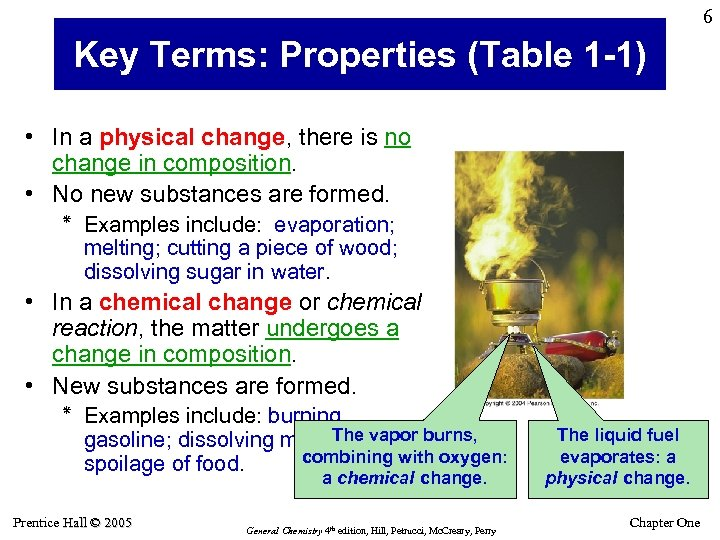6 Key Terms: Properties (Table 1 -1) • In a physical change, there is no change in composition. • No new substances are formed. ٭ Examples include: evaporation; melting; cutting a piece of wood; dissolving sugar in water. • In a chemical change or chemical reaction, the matter undergoes a change in composition. • New substances are formed. ٭ Examples include: burning gasoline; dissolving metal. Theacid; burns, in vapor combining with oxygen: spoilage of food. a chemical change. Prentice Hall © 2005 General Chemistry 4 th edition, Hill, Petrucci, Mc. Creary, Perry The liquid fuel evaporates: a physical change. Chapter One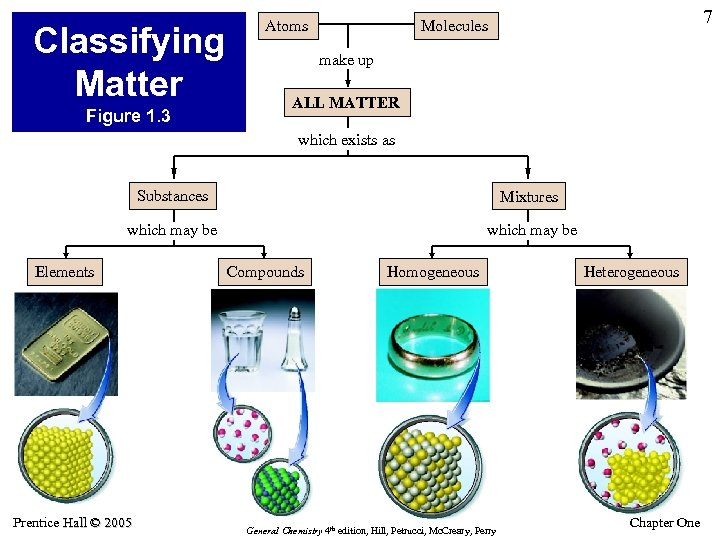Classifying Matter Figure 1. 3 Atoms 7 Molecules make up ALL MATTER which exists as Substances Mixtures which may be Elements Prentice Hall © 2005 Compounds Homogeneous General Chemistry 4 th edition, Hill, Petrucci, Mc. Creary, Perry Heterogeneous Chapter One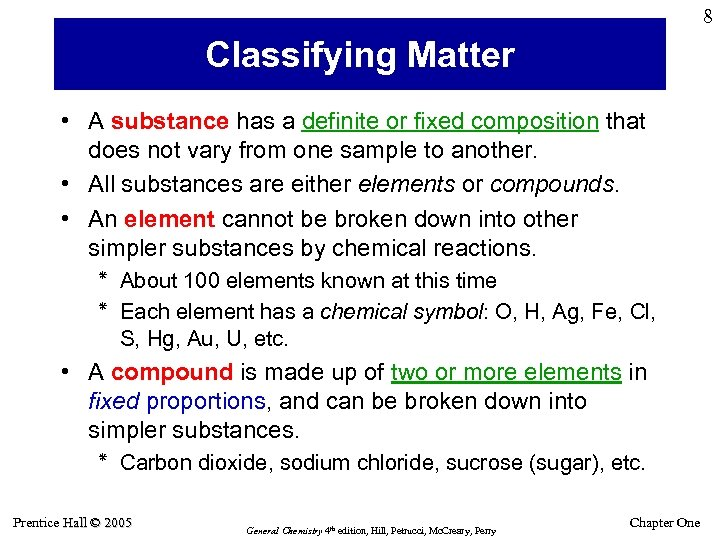8 Classifying Matter • A substance has a definite or fixed composition that does not vary from one sample to another. • All substances are either elements or compounds. • An element cannot be broken down into other simpler substances by chemical reactions. ٭ About 100 elements known at this time ٭ Each element has a chemical symbol: O, H, Ag, Fe, Cl, S, Hg, Au, U, etc. • A compound is made up of two or more elements in fixed proportions, and can be broken down into simpler substances. ٭ Carbon dioxide, sodium chloride, sucrose (sugar), etc. Prentice Hall © 2005 General Chemistry 4 th edition, Hill, Petrucci, Mc. Creary, Perry Chapter One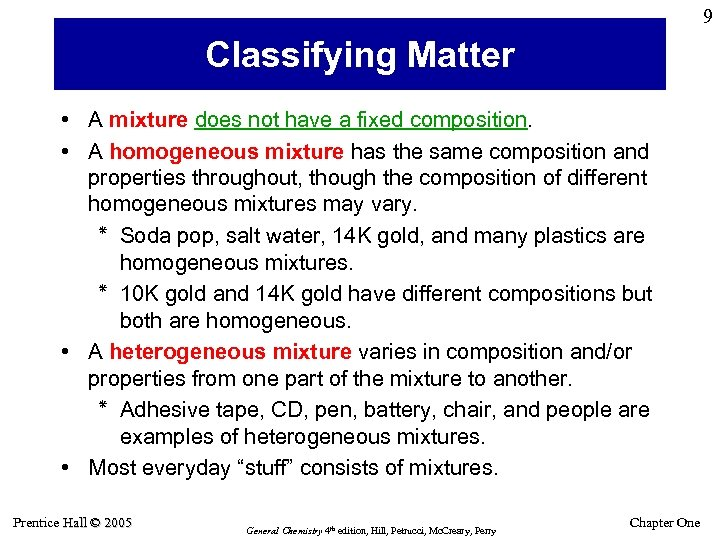9 Classifying Matter • A mixture does not have a fixed composition. • A homogeneous mixture has the same composition and properties throughout, though the composition of different homogeneous mixtures may vary. ٭ Soda pop, salt water, 14 K gold, and many plastics are homogeneous mixtures. 01 ٭ K gold and 14 K gold have different compositions but both are homogeneous. • A heterogeneous mixture varies in composition and/or properties from one part of the mixture to another. ٭ Adhesive tape, CD, pen, battery, chair, and people are examples of heterogeneous mixtures. • Most everyday “stuff” consists of mixtures. Prentice Hall © 2005 General Chemistry 4 th edition, Hill, Petrucci, Mc. Creary, Perry Chapter One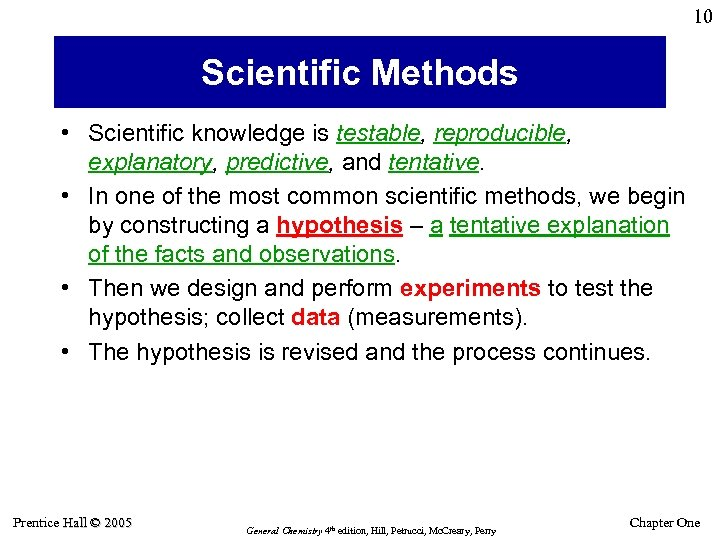10 Scientific Methods • Scientific knowledge is testable, reproducible, explanatory, predictive, and tentative. • In one of the most common scientific methods, we begin by constructing a hypothesis – a tentative explanation of the facts and observations. • Then we design and perform experiments to test the hypothesis; collect data (measurements). • The hypothesis is revised and the process continues. Prentice Hall © 2005 General Chemistry 4 th edition, Hill, Petrucci, Mc. Creary, Perry Chapter One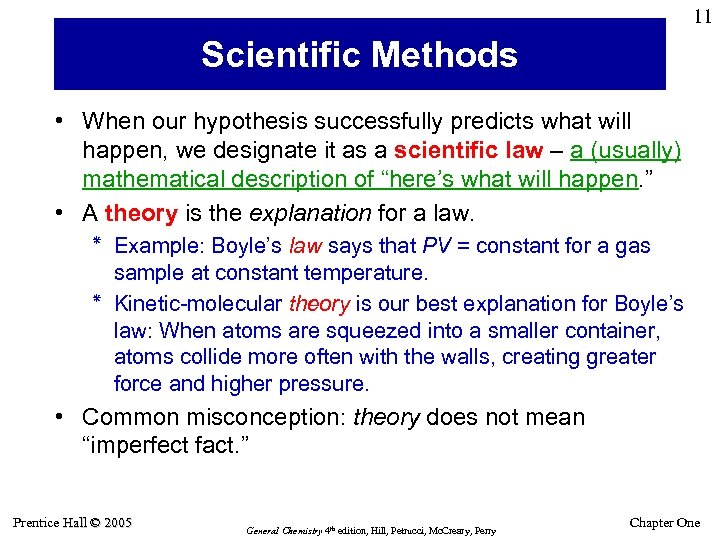11 Scientific Methods • When our hypothesis successfully predicts what will happen, we designate it as a scientific law – a (usually) mathematical description of “here’s what will happen. ” • A theory is the explanation for a law. ٭ Example: Boyle’s law says that PV = constant for a gas sample at constant temperature. ٭ Kinetic-molecular theory is our best explanation for Boyle’s law: When atoms are squeezed into a smaller container, atoms collide more often with the walls, creating greater force and higher pressure. • Common misconception: theory does not mean “imperfect fact. ” Prentice Hall © 2005 General Chemistry 4 th edition, Hill, Petrucci, Mc. Creary, Perry Chapter One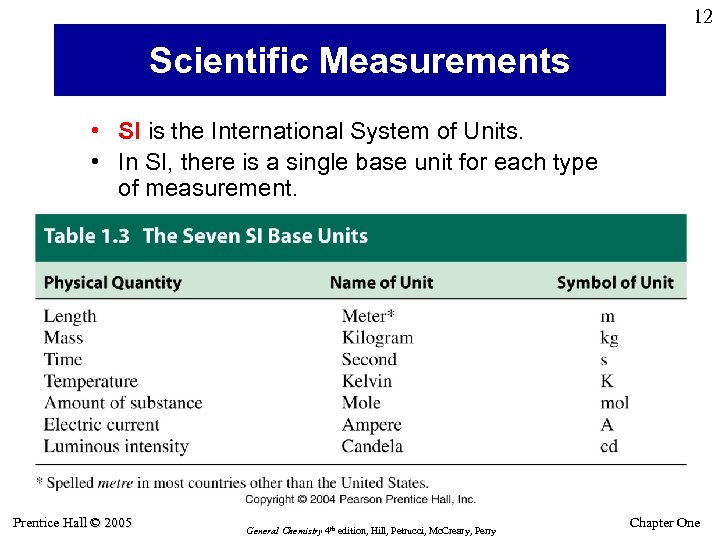12 Scientific Measurements • SI is the International System of Units. • In SI, there is a single base unit for each type of measurement. Prentice Hall © 2005 General Chemistry 4 th edition, Hill, Petrucci, Mc. Creary, Perry Chapter One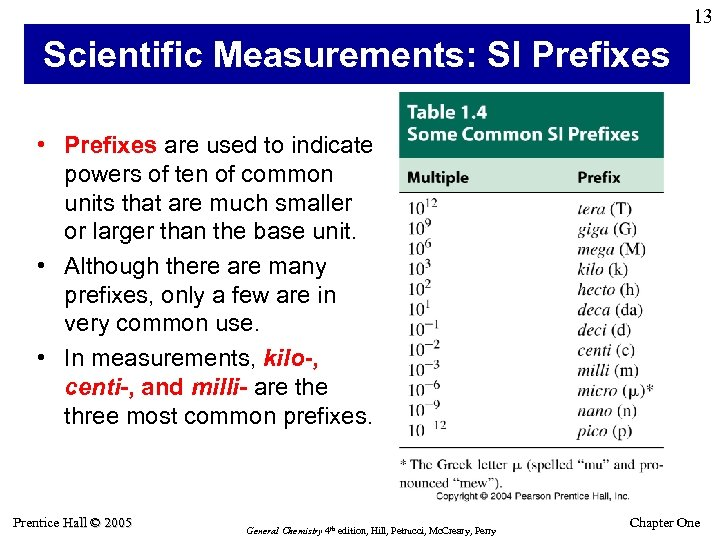13 Scientific Measurements: SI Prefixes • Prefixes are used to indicate powers of ten of common units that are much smaller or larger than the base unit. • Although there are many prefixes, only a few are in very common use. • In measurements, kilo-, centi-, and milli- are three most common prefixes. Prentice Hall © 2005 General Chemistry 4 th edition, Hill, Petrucci, Mc. Creary, Perry Chapter One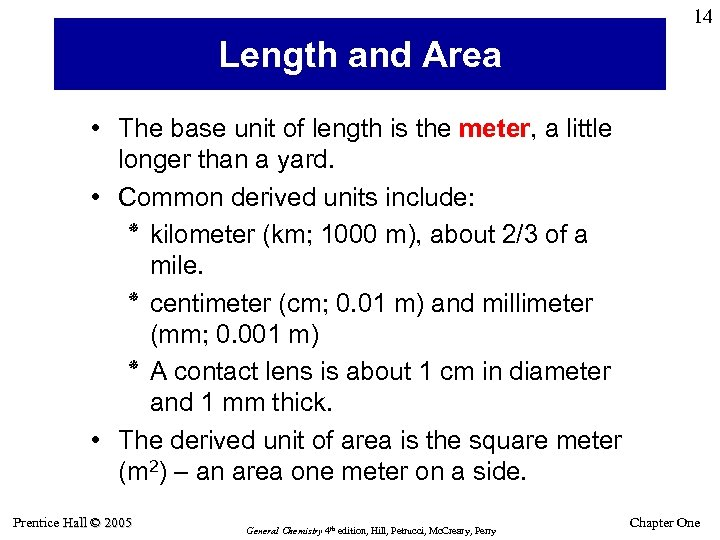14 Length and Area • The base unit of length is the meter, a little longer than a yard. • Common derived units include: ٭ kilometer (km; 1000 m), about 2/3 of a mile. ٭ centimeter (cm; 0. 01 m) and millimeter (mm; 0. 001 m) ٭ A contact lens is about 1 cm in diameter and 1 mm thick. • The derived unit of area is the square meter (m 2) – an area one meter on a side. Prentice Hall © 2005 General Chemistry 4 th edition, Hill, Petrucci, Mc. Creary, Perry Chapter One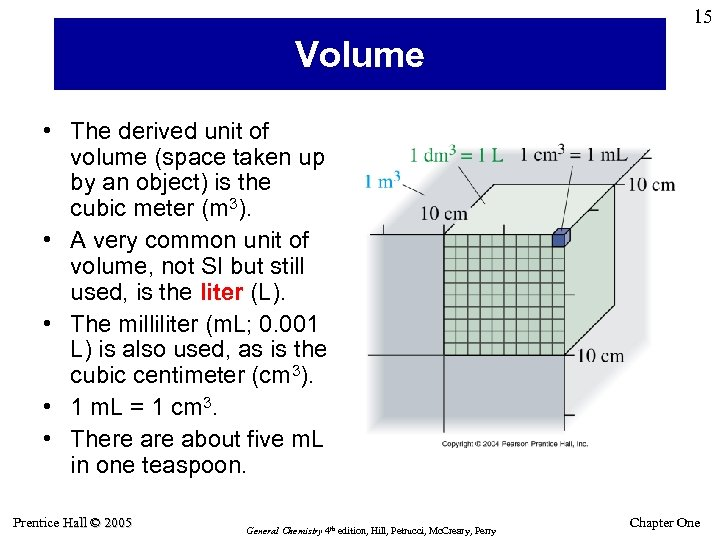15 Volume • The derived unit of volume (space taken up by an object) is the cubic meter (m 3). • A very common unit of volume, not SI but still used, is the liter (L). • The milliliter (m. L; 0. 001 L) is also used, as is the cubic centimeter (cm 3). • 1 m. L = 1 cm 3. • There about five m. L in one teaspoon. Prentice Hall © 2005 General Chemistry 4 th edition, Hill, Petrucci, Mc. Creary, Perry Chapter One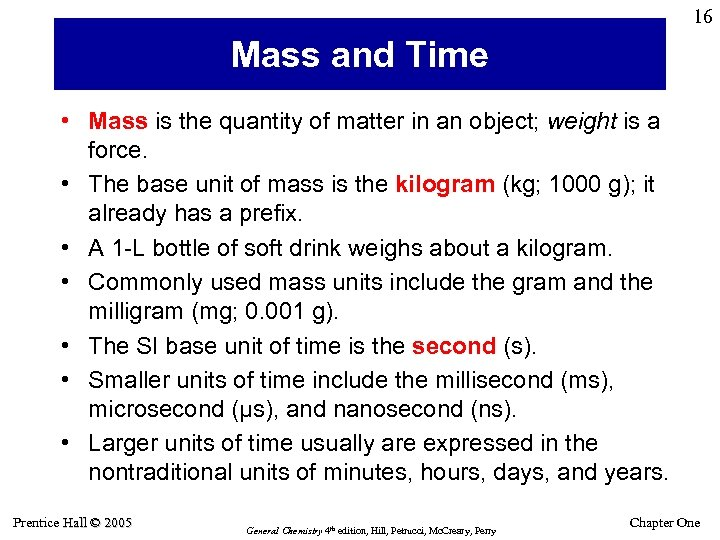16 Mass and Time • Mass is the quantity of matter in an object; weight is a force. • The base unit of mass is the kilogram (kg; 1000 g); it already has a prefix. • A 1 -L bottle of soft drink weighs about a kilogram. • Commonly used mass units include the gram and the milligram (mg; 0. 001 g). • The SI base unit of time is the second (s). • Smaller units of time include the millisecond (ms), microsecond (µs), and nanosecond (ns). • Larger units of time usually are expressed in the nontraditional units of minutes, hours, days, and years. Prentice Hall © 2005 General Chemistry 4 th edition, Hill, Petrucci, Mc. Creary, Perry Chapter One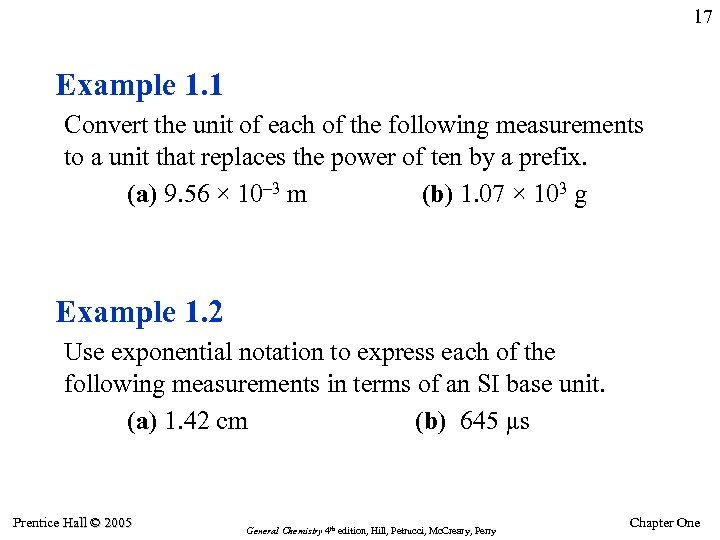17 Example 1. 1 Convert the unit of each of the following measurements to a unit that replaces the power of ten by a prefix. (a) 9. 56 × 10– 3 m (b) 1. 07 × 103 g Example 1. 2 Use exponential notation to express each of the following measurements in terms of an SI base unit. (a) 1. 42 cm (b) 645 µs Prentice Hall © 2005 General Chemistry 4 th edition, Hill, Petrucci, Mc. Creary, Perry Chapter One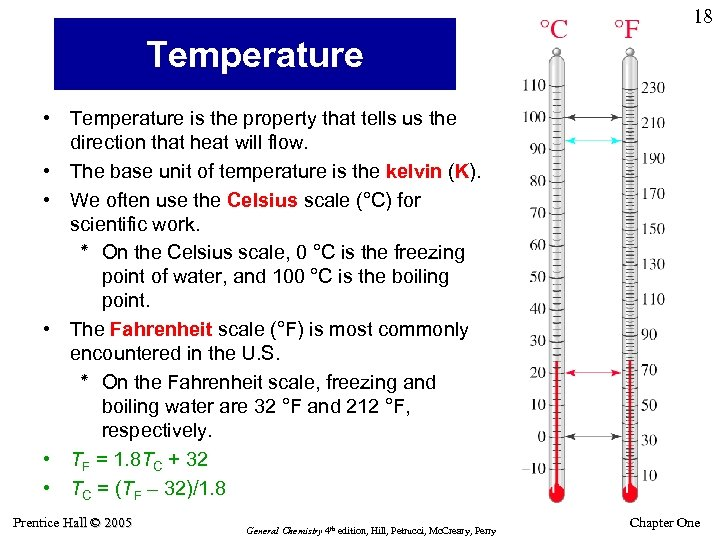18 Temperature • Temperature is the property that tells us the direction that heat will flow. • The base unit of temperature is the kelvin (K). • We often use the Celsius scale (°C) for scientific work. ٭ On the Celsius scale, 0 °C is the freezing point of water, and 100 °C is the boiling point. • The Fahrenheit scale (°F) is most commonly encountered in the U. S. ٭ On the Fahrenheit scale, freezing and boiling water are 32 °F and 212 °F, respectively. • TF = 1. 8 TC + 32 • TC = (TF – 32)/1. 8 Prentice Hall © 2005 General Chemistry 4 th edition, Hill, Petrucci, Mc. Creary, Perry Chapter One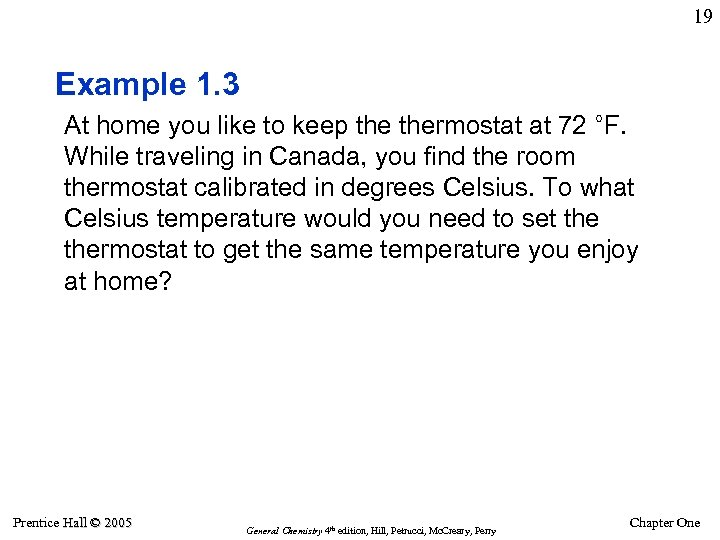19 Example 1. 3 At home you like to keep thermostat at 72 °F. While traveling in Canada, you find the room thermostat calibrated in degrees Celsius. To what Celsius temperature would you need to set thermostat to get the same temperature you enjoy at home? Prentice Hall © 2005 General Chemistry 4 th edition, Hill, Petrucci, Mc. Creary, Perry Chapter One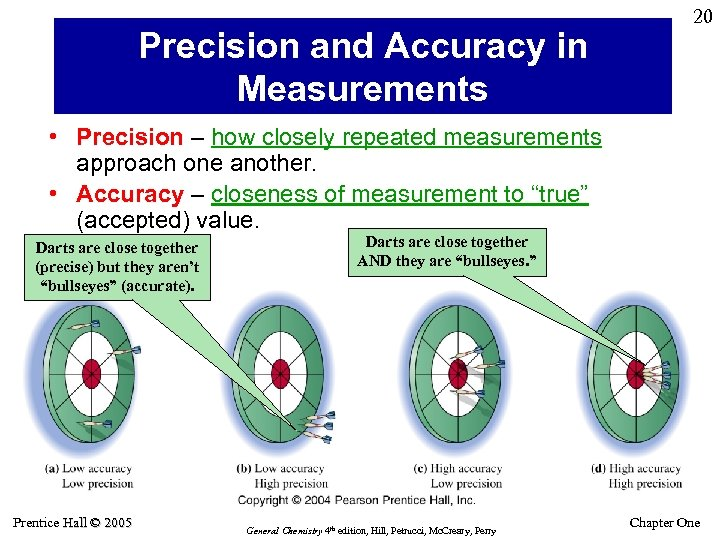Precision and Accuracy in Measurements 20 • Precision – how closely repeated measurements approach one another. • Accuracy – closeness of measurement to “true” (accepted) value. Darts are close together (precise) but they aren’t “bullseyes” (accurate). Prentice Hall © 2005 Darts are close together AND they are “bullseyes. ” General Chemistry 4 th edition, Hill, Petrucci, Mc. Creary, Perry Chapter One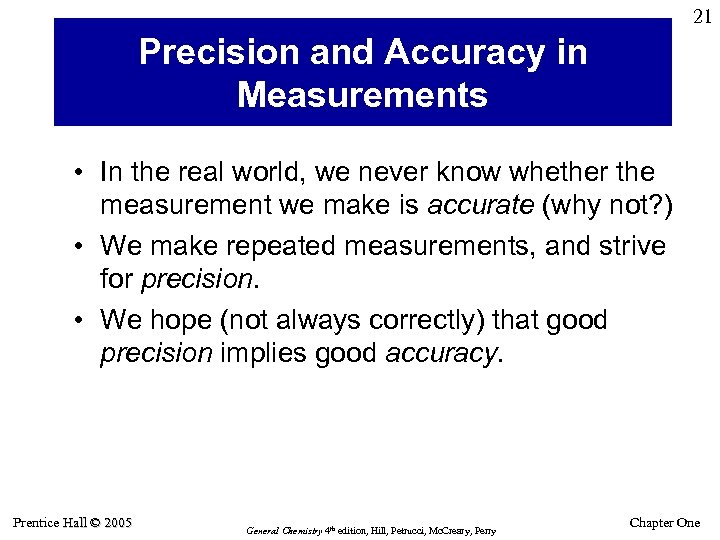21 Precision and Accuracy in Measurements • In the real world, we never know whether the measurement we make is accurate (why not? ) • We make repeated measurements, and strive for precision. • We hope (not always correctly) that good precision implies good accuracy. Prentice Hall © 2005 General Chemistry 4 th edition, Hill, Petrucci, Mc. Creary, Perry Chapter One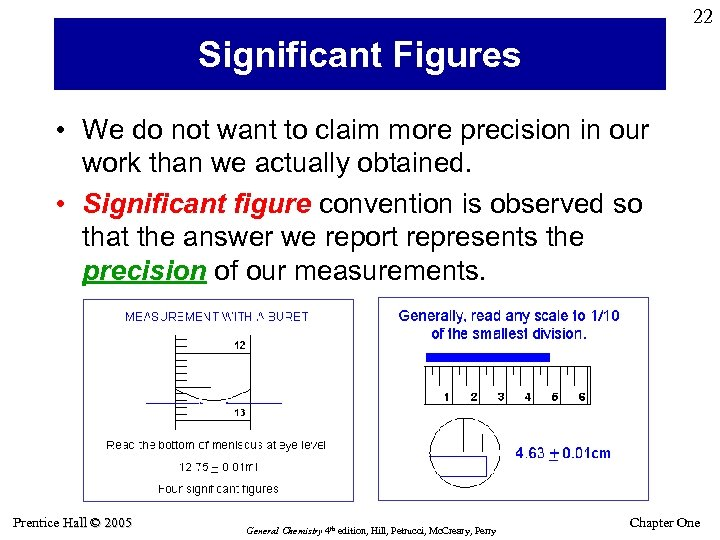22 Significant Figures • We do not want to claim more precision in our work than we actually obtained. • Significant figure convention is observed so that the answer we report represents the precision of our measurements. Prentice Hall © 2005 General Chemistry 4 th edition, Hill, Petrucci, Mc. Creary, Perry Chapter One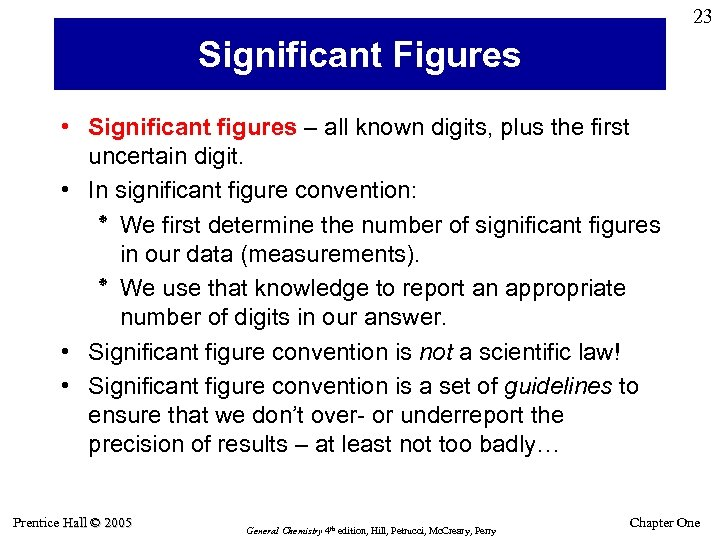23 Significant Figures • Significant figures – all known digits, plus the first uncertain digit. • In significant figure convention: ٭ We first determine the number of significant figures in our data (measurements). ٭ We use that knowledge to report an appropriate number of digits in our answer. • Significant figure convention is not a scientific law! • Significant figure convention is a set of guidelines to ensure that we don’t over- or underreport the precision of results – at least not too badly… Prentice Hall © 2005 General Chemistry 4 th edition, Hill, Petrucci, Mc. Creary, Perry Chapter One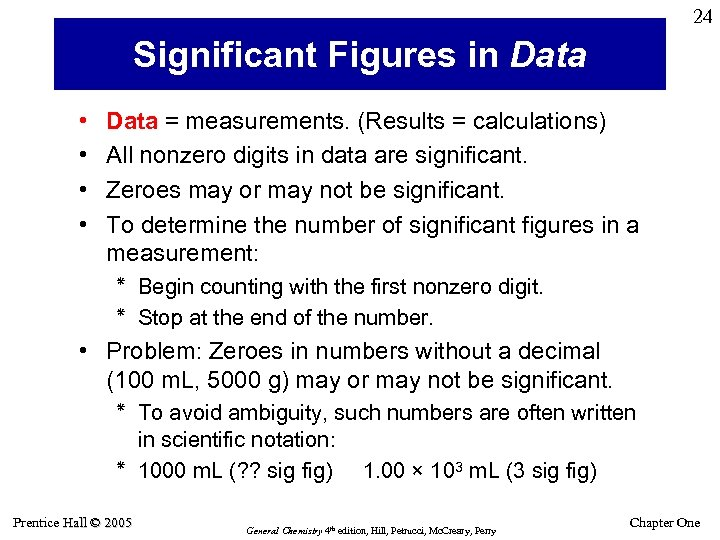24 Significant Figures in Data • • Data = measurements. (Results = calculations) All nonzero digits in data are significant. Zeroes may or may not be significant. To determine the number of significant figures in a measurement: ٭ Begin counting with the first nonzero digit. ٭ Stop at the end of the number. • Problem: Zeroes in numbers without a decimal (100 m. L, 5000 g) may or may not be significant. ٭ To avoid ambiguity, such numbers are often written in scientific notation: 0001 ٭ m. L (? ? sig fig) 1. 00 × 103 m. L (3 sig fig) Prentice Hall © 2005 General Chemistry 4 th edition, Hill, Petrucci, Mc. Creary, Perry Chapter One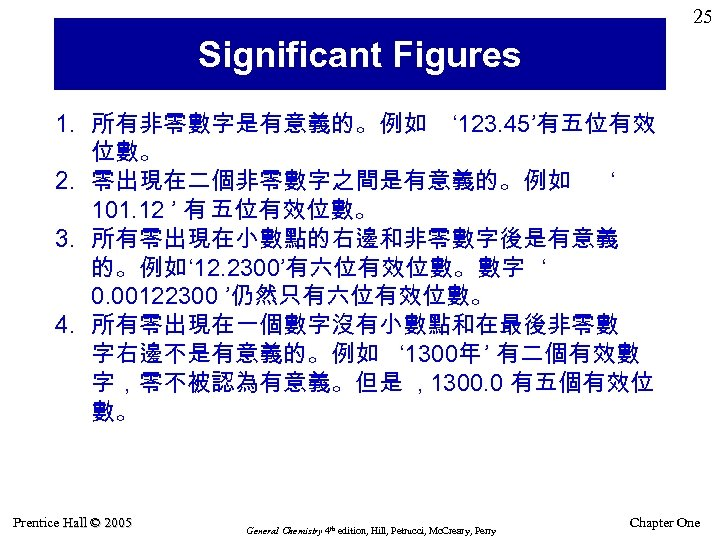25 Significant Figures 1. 所有非零數字是有意義的。例如 ‘ 123. 45’有五位有效 位數。 2. 零出現在二個非零數字之間是有意義的。例如 ‘ 101. 12 ’ 有 五位有效位數。 3. 所有零出現在小數點的右邊和非零數字後是有意義 的。例如‘ 12. 2300’有六位有效位數。數字 ‘ 0. 00122300 ’仍然只有六位有效位數。 4. 所有零出現在一個數字沒有小數點和在最後非零數 字右邊不是有意義的。例如 ‘ 1300年 ’ 有二個有效數 字，零不被認為有意義。但是 , 1300. 0 有五個有效位 數。 Prentice Hall © 2005 General Chemistry 4 th edition, Hill, Petrucci, Mc. Creary, Perry Chapter One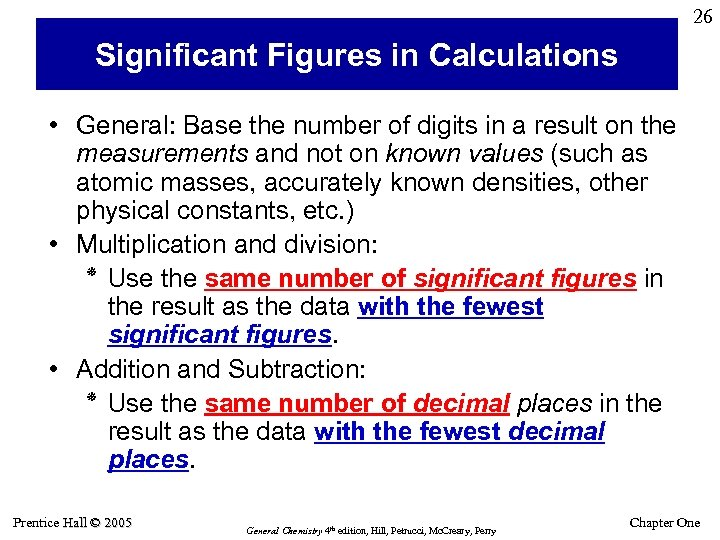26 Significant Figures in Calculations • General: Base the number of digits in a result on the measurements and not on known values (such as atomic masses, accurately known densities, other physical constants, etc. ) • Multiplication and division: ٭ Use the same number of significant figures in the result as the data with the fewest significant figures. • Addition and Subtraction: ٭ Use the same number of decimal places in the result as the data with the fewest decimal places. Prentice Hall © 2005 General Chemistry 4 th edition, Hill, Petrucci, Mc. Creary, Perry Chapter One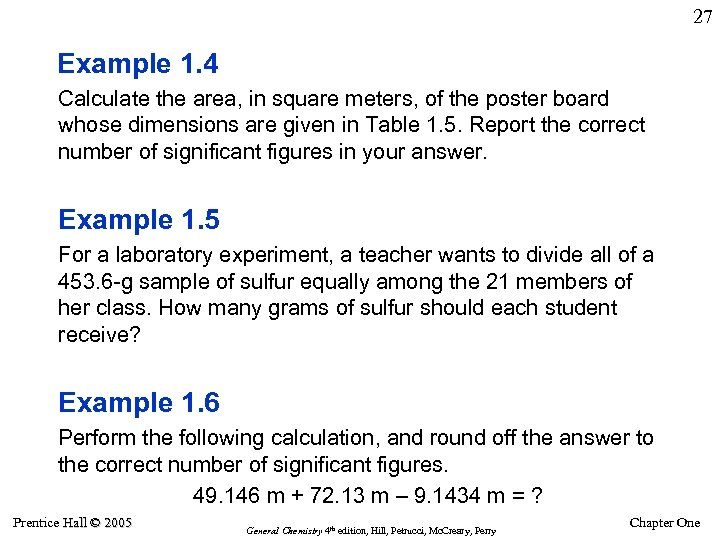27 Example 1. 4 Calculate the area, in square meters, of the poster board whose dimensions are given in Table 1. 5. Report the correct number of significant figures in your answer. Example 1. 5 For a laboratory experiment, a teacher wants to divide all of a 453. 6 -g sample of sulfur equally among the 21 members of her class. How many grams of sulfur should each student receive? Example 1. 6 Perform the following calculation, and round off the answer to the correct number of significant figures. 49. 146 m + 72. 13 m – 9. 1434 m = ? Prentice Hall © 2005 General Chemistry 4 th edition, Hill, Petrucci, Mc. Creary, Perry Chapter One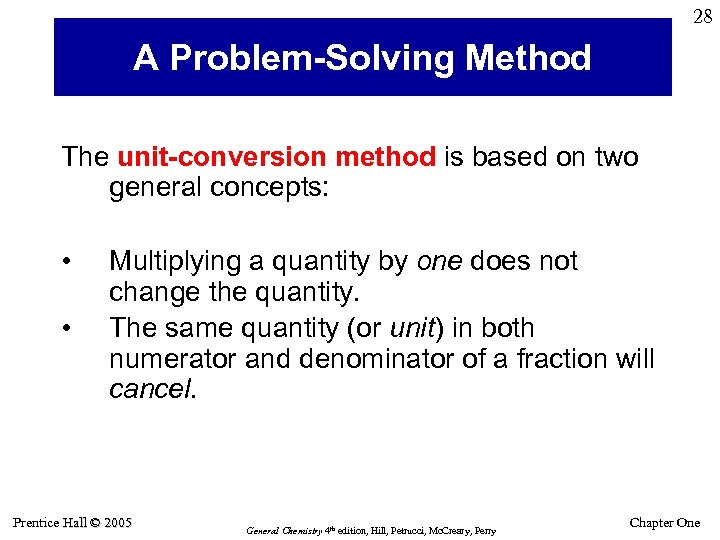28 A Problem-Solving Method The unit-conversion method is based on two general concepts: • • Multiplying a quantity by one does not change the quantity. The same quantity (or unit) in both numerator and denominator of a fraction will cancel. Prentice Hall © 2005 General Chemistry 4 th edition, Hill, Petrucci, Mc. Creary, Perry Chapter One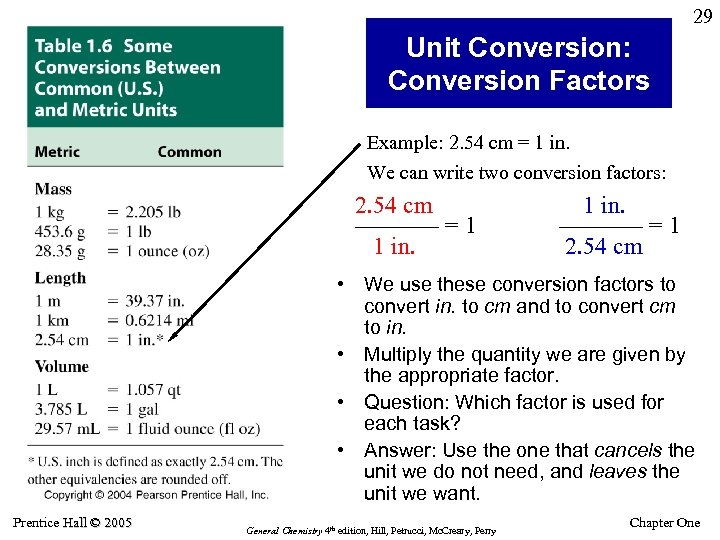29 Unit Conversion: Conversion Factors Example: 2. 54 cm = 1 in. We can write two conversion factors: 2. 54 cm ––––––– = 1 1 in. ––––––– = 1 2. 54 cm • We use these conversion factors to convert in. to cm and to convert cm to in. • Multiply the quantity we are given by the appropriate factor. • Question: Which factor is used for each task? • Answer: Use the one that cancels the unit we do not need, and leaves the unit we want. Prentice Hall © 2005 General Chemistry 4 th edition, Hill, Petrucci, Mc. Creary, Perry Chapter One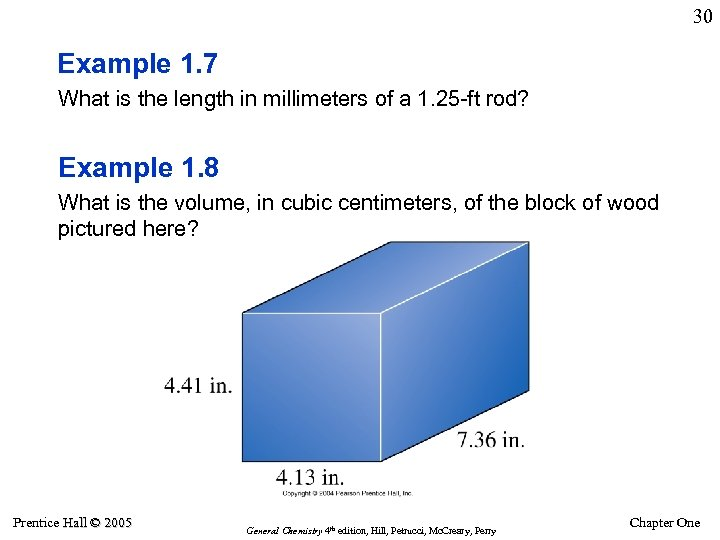30 Example 1. 7 What is the length in millimeters of a 1. 25 -ft rod? Example 1. 8 What is the volume, in cubic centimeters, of the block of wood pictured here? Prentice Hall © 2005 General Chemistry 4 th edition, Hill, Petrucci, Mc. Creary, Perry Chapter One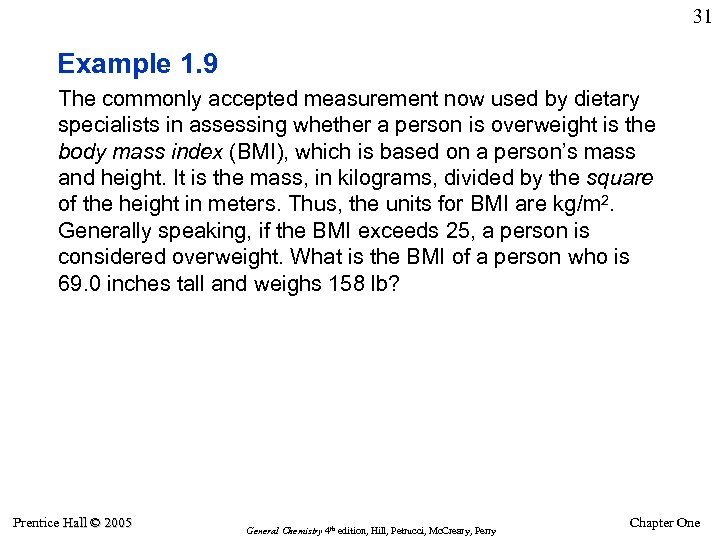31 Example 1. 9 The commonly accepted measurement now used by dietary specialists in assessing whether a person is overweight is the body mass index (BMI), which is based on a person’s mass and height. It is the mass, in kilograms, divided by the square of the height in meters. Thus, the units for BMI are kg/m 2. Generally speaking, if the BMI exceeds 25, a person is considered overweight. What is the BMI of a person who is 69. 0 inches tall and weighs 158 lb? Prentice Hall © 2005 General Chemistry 4 th edition, Hill, Petrucci, Mc. Creary, Perry Chapter One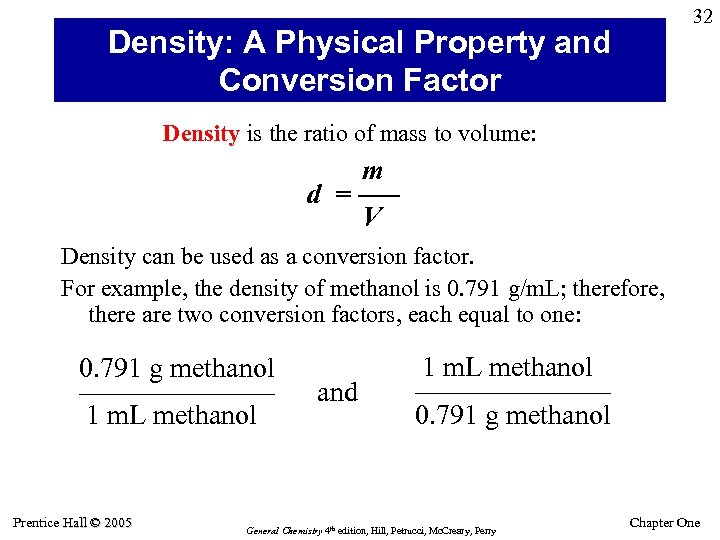32 Density: A Physical Property and Conversion Factor Density is the ratio of mass to volume: m d = ––– V Density can be used as a conversion factor. For example, the density of methanol is 0. 791 g/m. L; therefore, there are two conversion factors, each equal to one: 0. 791 g methanol ––––––– 1 m. L methanol Prentice Hall © 2005 and 1 m. L methanol ––––––– 0. 791 g methanol General Chemistry 4 th edition, Hill, Petrucci, Mc. Creary, Perry Chapter One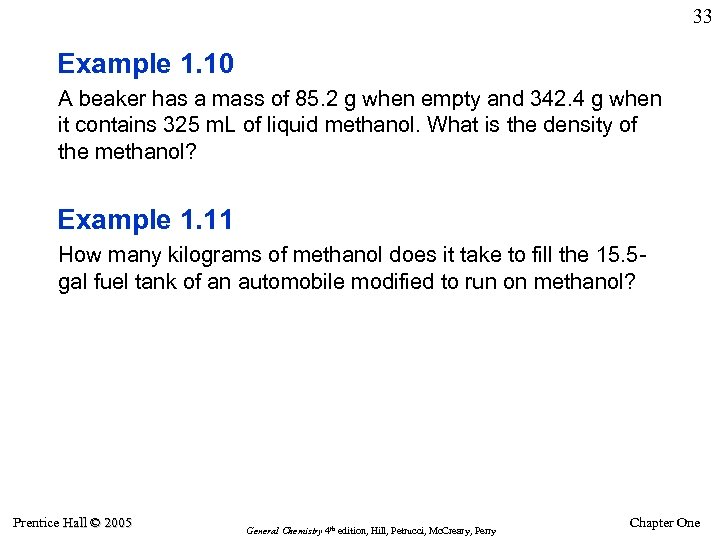33 Example 1. 10 A beaker has a mass of 85. 2 g when empty and 342. 4 g when it contains 325 m. L of liquid methanol. What is the density of the methanol? Example 1. 11 How many kilograms of methanol does it take to fill the 15. 5 gal fuel tank of an automobile modified to run on methanol? Prentice Hall © 2005 General Chemistry 4 th edition, Hill, Petrucci, Mc. Creary, Perry Chapter One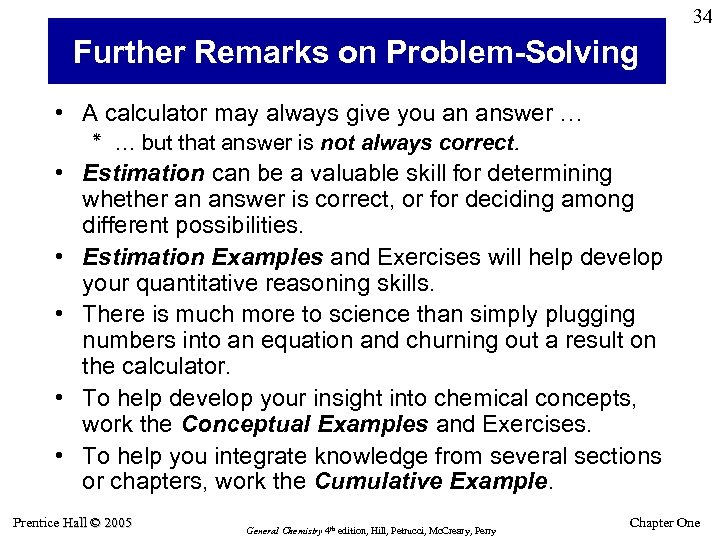34 Further Remarks on Problem-Solving • A calculator may always give you an answer … … ٭ but that answer is not always correct. • Estimation can be a valuable skill for determining whether an answer is correct, or for deciding among different possibilities. • Estimation Examples and Exercises will help develop your quantitative reasoning skills. • There is much more to science than simply plugging numbers into an equation and churning out a result on the calculator. • To help develop your insight into chemical concepts, work the Conceptual Examples and Exercises. • To help you integrate knowledge from several sections or chapters, work the Cumulative Example. Prentice Hall © 2005 General Chemistry 4 th edition, Hill, Petrucci, Mc. Creary, Perry Chapter One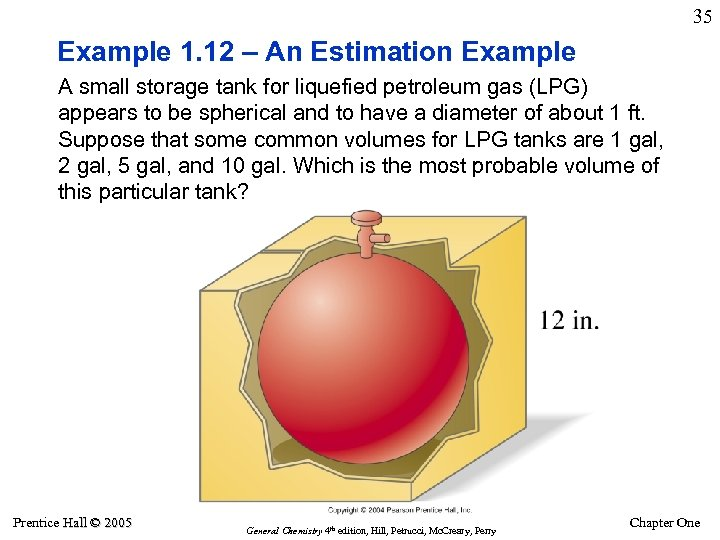35 Example 1. 12 – An Estimation Example A small storage tank for liquefied petroleum gas (LPG) appears to be spherical and to have a diameter of about 1 ft. Suppose that some common volumes for LPG tanks are 1 gal, 2 gal, 5 gal, and 10 gal. Which is the most probable volume of this particular tank? Prentice Hall © 2005 General Chemistry 4 th edition, Hill, Petrucci, Mc. Creary, Perry Chapter One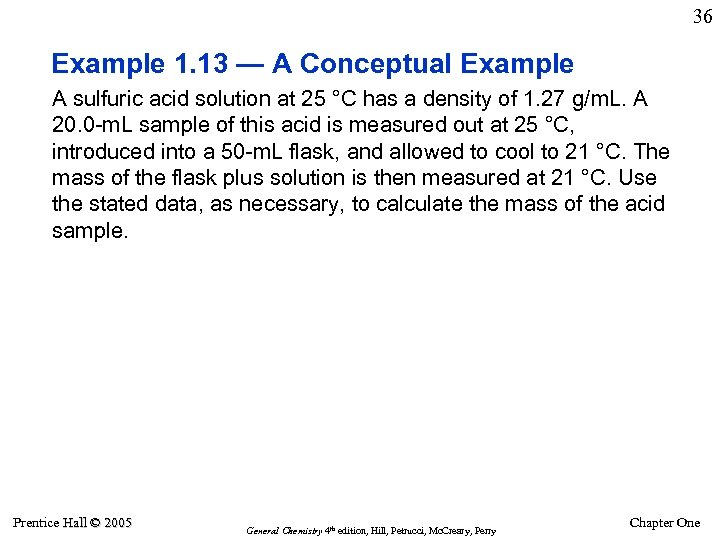36 Example 1. 13 — A Conceptual Example A sulfuric acid solution at 25 °C has a density of 1. 27 g/m. L. A 20. 0 -m. L sample of this acid is measured out at 25 °C, introduced into a 50 -m. L flask, and allowed to cool to 21 °C. The mass of the flask plus solution is then measured at 21 °C. Use the stated data, as necessary, to calculate the mass of the acid sample. Prentice Hall © 2005 General Chemistry 4 th edition, Hill, Petrucci, Mc. Creary, Perry Chapter One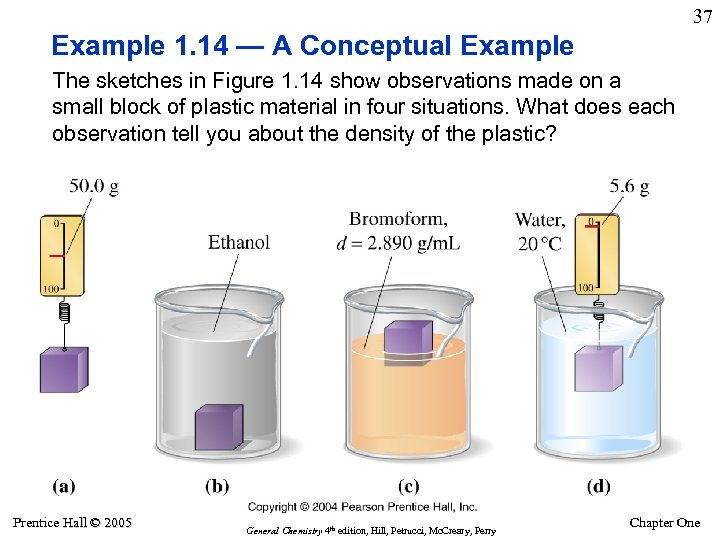37 Example 1. 14 — A Conceptual Example The sketches in Figure 1. 14 show observations made on a small block of plastic material in four situations. What does each observation tell you about the density of the plastic? Prentice Hall © 2005 General Chemistry 4 th edition, Hill, Petrucci, Mc. Creary, Perry Chapter One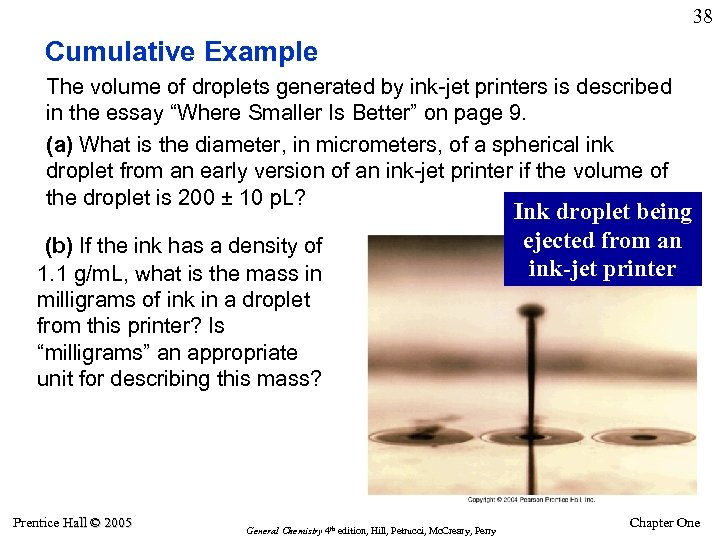38 Cumulative Example The volume of droplets generated by ink-jet printers is described in the essay “Where Smaller Is Better” on page 9. (a) What is the diameter, in micrometers, of a spherical ink droplet from an early version of an ink-jet printer if the volume of the droplet is 200 ± 10 p. L? (b) If the ink has a density of 1. 1 g/m. L, what is the mass in milligrams of ink in a droplet from this printer? Is “milligrams” an appropriate unit for describing this mass? Prentice Hall © 2005 General Chemistry 4 th edition, Hill, Petrucci, Mc. Creary, Perry Ink droplet being ejected from an ink-jet printer Chapter One